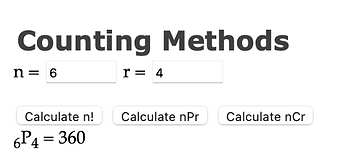## Chapter 5 - Day 8

##### Learning Targets
• Use the multiplication counting principle to determine the number of ways to complete a process involving several steps.

• Use factorials to count the number of permutations of a group of individuals.

• Compute the number of permutations of n individuals taken r at a time.

Experience First

The purpose of today’s activity is for students to be able to count (or calculate) the total number of ways to arrange items. The activity starts with them listing out all possibilities in question #1, which becomes impossible for them to do in #2, and necessitates a new tool (The Multiplication Counting Principle). In questions #3 and #4, we are trying to get students calculating factorials and permutations without ever having heard these terms before. The formal vocabulary and notation come in the debrief of the activity.

Formalize Later

For the last question in the activity, be sure to emphasize that for permutations the order matters. 1234 is a different password than 4321. Making this distinction now will help setup the learning in Lesson 5.8, where students calculate combinations because order doesn’t matter.

For calculating factorials and permutation, students can use calculators or the “Counting Methods” applet at www.statsmedic.com/applets.